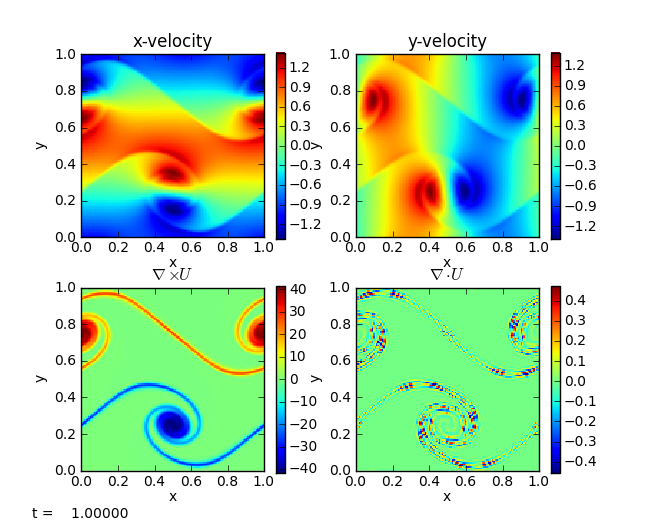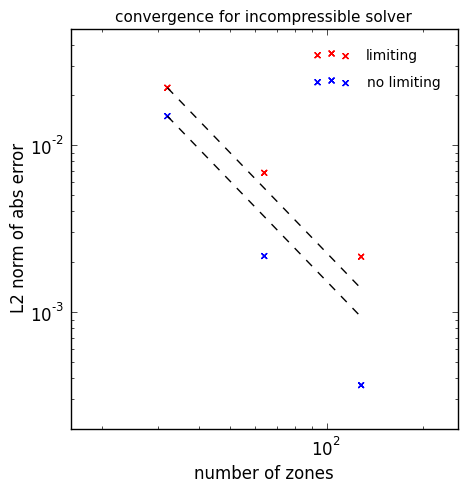# Incompressible hydrodynamics solver¶

pyro’s incompressible solver solves:

$\begin{split}\frac{\partial U}{\partial t} + U \cdot \nabla U + \nabla p &= 0 \\ \nabla \cdot U &= 0\end{split}$

The algorithm combines the Godunov/advection features used in the advection and compressible solver together with multigrid to enforce the divergence constraint on the velocities.

Here we implement a cell-centered approximate projection method for solving the incompressible equations. At the moment, only periodic BCs are supported.

The main parameters that affect this solver are:

• section: [driver]

option

value

description

cfl

0.8

• section: [incompressible]

option

value

description

limiter

2

limiter (0 = none, 1 = 2nd order, 2 = 4th order)

proj_type

2

what are we projecting? 1 includes -Gp term in U*

• section: [particles]

option

value

description

do_particles

0

particle_generator

grid

## Examples¶

### shear¶

The shear problem initializes a shear layer in a domain with doubly-periodic boundaries and looks at the development of two vortices as the shear layer rolls up. This problem was explored in a number of papers, for example, Bell, Colella, & Glaz (1989) and Martin & Colella (2000). This is run as:

./pyro.py incompressible shear inputs.shearThe vorticity panel (lower left) is what is usually shown in papers. Note that the velocity divergence is not zero—this is because we are using an approximate projection.

### convergence¶

The convergence test initializes a simple velocity field on a periodic unit square with known analytic solution. By evolving at a variety of resolutions and comparing to the analytic solution, we can measure the convergence rate of the algorithm. The particular set of initial conditions is from Minion (1996). Limiting can be disabled by adding incompressible.limiter=0 to the run command. The basic set of tests shown below are run as:

./pyro.py incompressible converge inputs.converge.32 vis.dovis=0
./pyro.py incompressible converge inputs.converge.64 vis.dovis=0
./pyro.py incompressible converge inputs.converge.128 vis.dovis=0


The error is measured by comparing with the analytic solution using the routine incomp_converge_error.py in analysis/.The dashed line is second order convergence. We see almost second order behavior with the limiters enabled and slightly better than second order with no limiting.

## Exercises¶

### Explorations¶

• Disable the MAC projection and run the converge problem—is the method still 2nd order?

• Disable all projections—does the solution still even try to preserve $$\nabla \cdot U = 0$$?

• Experiment with what is projected. Try projecting $$U_t$$ to see if that makes a difference.

### Extensions¶

• Switch the final projection from a cell-centered approximate projection to a nodal projection. This will require writing a new multigrid solver that operates on nodal data.

• Add viscosity to the system. This will require doing 2 parabolic solves (one for each velocity component). These solves will look like the diffusion operation, and will update the provisional velocity field.

• Switch to a variable density system. This will require adding a mass continuity equation that is advected and switching the projections to a variable-coeffient form (since ρ now enters).

## Going further¶

The incompressible algorithm presented here is a simplified version of the projection methods used in the Maestro low Mach number hydrodynamics code. Maestro can do variable-density incompressible, anelastic, and low Mach number stratified flows in stellar (and terrestrial) environments in close hydrostatic equilibrium.